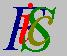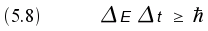Mauro Murzi's pages on Philosophy of Science - Quantum mechanics
Applications of the indeterminacy principleMean values Links# [5. Heisenberg indeterminacy principle.]

## Applications of the indeterminacy principle.

We can use Heisenberg indeterminacy principle to understand why a quantum particle is able to escape from a potential well. The position q of the particle inside a potential well is strongly localized, thus the indetermination of its momentum p is high. Since the kinetic energy T of the particle is a function of its momentum p (T = p²/2m), the indetermination of the energy is high too. As a consequence of this indetermination, the particle can have enough energy to escape.

Another application of the indeterminacy principle concerns the zero-point energy. A quantum particle cannot be completely at rest, because in such case the indetermination of its position and momentum will be zero. This is true also for a particle at absolute zero. In classical physics, a particle at absolute zero has no energy at all; in quantum mechanics, a particle at absolute zero is not at rest, so it has a residual energy.

The indeterminacy principle is applicable also to energy E and time t:When applied to the electromagnetic field, formula (5.8) states that also in empty space the electromagnetic field has an energy, called the vacuum energy. Given the equivalence between energy and mass, the vacuum energy can manifests itself creating couples of short-lived particles and anti-particles, which disappear in a time interval t determined by equation (5.8).Mean values Links